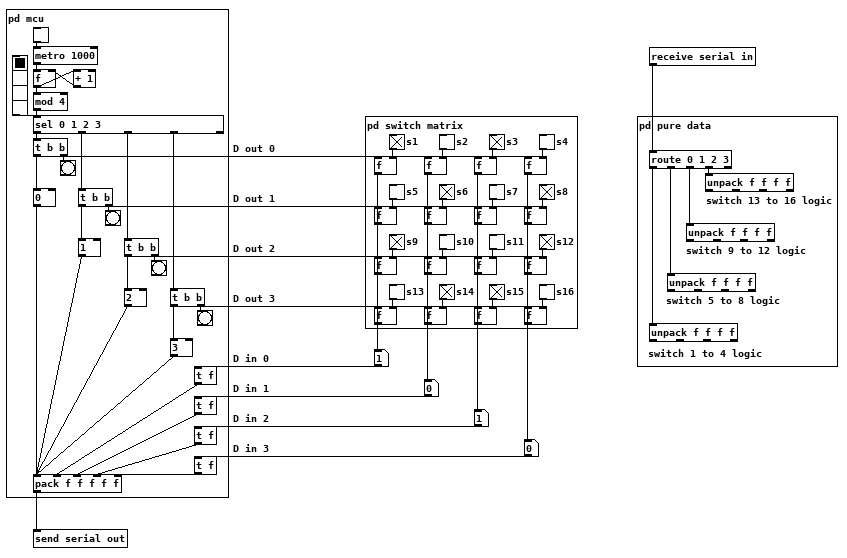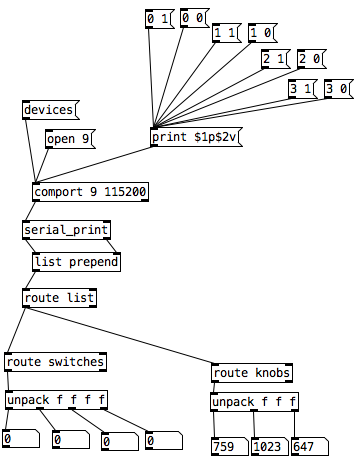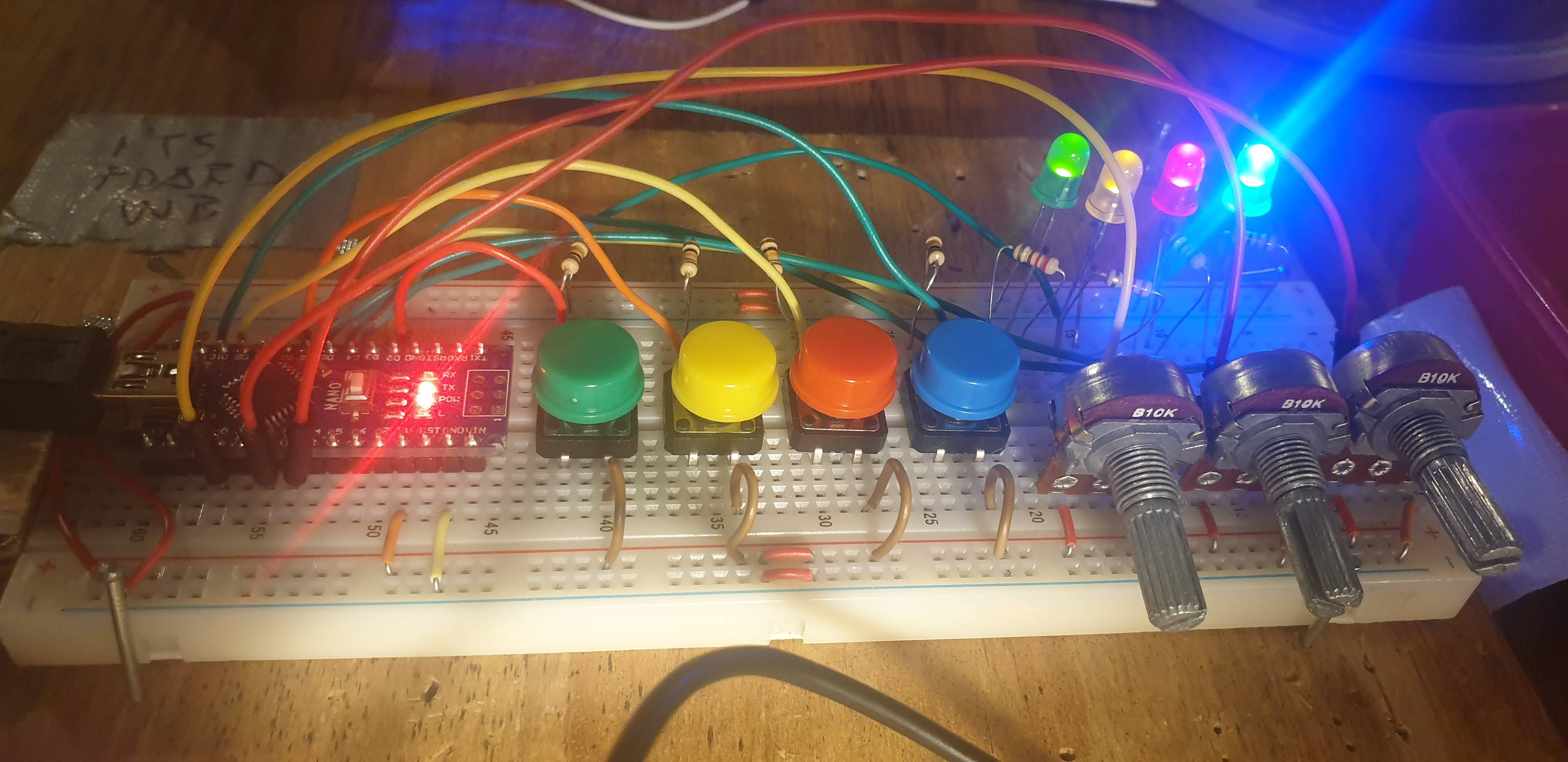• ### Having lots of switches into Pd

I have using four arduino uno in parallel with the Pduino library, using most of the digital and analog pins. As inputs. I have experienced many glitches with this setup and I have been OK with that since I am exploring and experimenting. But now I need to clean it up a bit.

What alternatives may there be instead of using arduino? Are there better solutions?

When using multiple arduinos, how to avoid problems?

Matrix switches... is this a good idea? To keep the arduino count low.

Thanks

• Posts 24 | Views 663
• @cfry What makes it hard to adapt to your scenerio? Just add more rows and/or columns to suit, adding emitter followers or cmos buffers on the ins and outs would not hurt, but you may not need them, I do not know the specs of the arduinos or your number of switches so I can not say for certain.

Perhaps putting everything into pd terms would help? switchmatrix.pdThe one caveat is that I have no idea if the mcu (arduino) can send things as lists or if it is just a serial stream of floats, no difficult to deal with the stream if that is the case anyways. This should show you the logic of the switch matrix and the MCUs code and allow some experimentation, at the very least it should make our communication more efficient?

• @cfry note that I have created two abstractions to simplify the communication between the Arduino and Pd. You can find them here. With these you don't need to do all the Pd patching plus you can use Arduino's `Serial.print()` function. Both abstractions are vanilla.

• @alexandros could you give an arduino code example on how to use digital in and out at the same time?

• @oid thanks for that pretty example patch. It made me really understand the whole concept and flow.

• Since you went through my tutorial and got some code working, can you share what you already have? Then I can suggest changes to make it work with [serial_print].

• @alexandros

Here is the working Arduino code, (which is basically a straight copy from "project 8", but I wrote it while reading the tutorial)

``````// setup for 6 analog in och 12 digital in (pullup to be added).
//intended as experimental template. use pd patch
//made with tutorial Arduino for Pd'ers, project 8

byte myArray;

void setup()
{
for(int i = 2; i < 14; i++)
pinMode(i, INPUT);
Serial.begin(9600);
}

void loop()
{
myArray = 0xc0;
int index = 1;

for(int i = 0; i < 6; i++){
unsigned int knob = analogRead (i);
myArray[index++] = knob & 0x007f;
myArray[index++] = knob >> 7;
}
for(int i = 2; i < 14; i++)

Serial.write(myArray, 25);
}
``````

Then I tried to incorporate project 1 (blink):

``````// set a variable to hold the ledddddddz pin number
int led = 13;

void setup()
{
//  st pin 13 as output, to light up the LED
// whenever it is told so from Pd
pinMode(led, OUTPUT);
// start the serial communication o the Arduino
// and Pd can communicate with each other
Serial.begin(9600);
}

void loop()
{
while(Serial.available()){
byte ledState = Serial.read() - '0';
digitalWrite(led, ledState);
}
}
``````

but when I tried to edit "blink" to work with several outputs and merge this in the project 8 code, I decided that I do not have enough understanding of the arduino code to pull it through. What is needed is, I think, another "for()" combined with digitalWrite() inside void loop()

Note: My plan now is to (still) use several Arduinos but with your code and abstractions (instead of the Pdunio). I will wait with the matrix switch setup since I have to take this in steps in order to have control of it.
This means that I am up and running, unless I want to use digital input and output from the same Arduino at the same time. I figure it would be great to have that sorted out beforehand so it works when I need it.

Thanks a lot.

• I would suggest to not mix the analog values with the digital ones. The first code could be changed to the following (using Serial.print() with the [serial_print] abstraction):

``````void setup()
{
for(int i = 2; i < 14; i++)
pinMode(i, INPUT);
Serial.begin(115200); // perhaps use a faster baud rate
}

void loop()
{
Serial.print("knobs"); // use "knobs" as a keyword so you can receive
// the knob values as a list with a [r knobs] in Pd
for(int i = 0; i < 6; i++){
unsigned int knob = analogRead (i);
Serial.print(" "); // first print a white space to separate the "knob" keyword from the values
// and the values from each other
Serial.print(knob); // then  print the actual knob value
}
Serial.println(); // finally print a newline character to denote end of data for keyword "knobs"

// the same technique applies to the switches too
// receive the switch values as a list with [r switches]
Serial.print("switches");
for(int i = 2; i < 14; i++) {
Serial.print(" ");
Serial.print(switchVal);
}
Serial.println();
}
``````

As for writing to several outputs you need to set which output you want to write to and then the value you want to write. Here's an example that writes to several different digital outputs:

``````int pin = 0;
int val = 0;
// some random pins
int pins = {3, 4, 5, 6];

void setup() {
for (int i = 0; i < 4; i++) {
pinMode(pins[i], OUTPUT);
digitalWrite(pins[i], LOW);
}

Serial.begin(115200);
}

void loop() {
if (Serial.available()) {
static int temp;
if (isDigit(in)) {
temp = temp * 10 + in - '0';
}
else if (in == 'p') {
pin = temp;
temp = 0;
}
else if (in == 'v')  {
val = temp;
temp = 0;
digitalWrite(pins[pin], val);
}
}
``````

With the code above you can send messages like this one `print \$1p\$2v` in Pd to the [comport] object. \$1 is the number of the pin you want to light up starting from 0 and incrementing by 1 (so the first pin used which is pin 3 in the Arduino code would be 0 in the Pd patch), and \$2 is the value, 0 or 1.
Note though that in the first code (and the code you posted), you're using all digital pins as inputs so there's no pin left to use as output. If you want to combine these two chunks of code you'll have to use less pins as inputs and leave some to be used as outputs.

• @alexandros thanks, it all works!

I merged the codes for in and outs and also changed so you can set up all of the pins with arrays (as you did in the latter code). Then you can set up the pins in any order.

Here is the Arduino code. Do you spot any errors I may have introduced?

``````//number of elements in array need to match for() cycles
int pinsIn = {2, 7, 10, 11};
int pinsAnalog = {0, 2, 3};

int pin = 0;
int val = 0;
// some random pins
int pinsOut = {3, 4, 5, 6};

void setup()
{
//set up a total of pins for input (has to match number of elements in array)
for(int i = 0; i < 4; i++)
pinMode(pinsIn[i], INPUT);

for (int i = 0; i < 4; i++) {
pinMode(pinsOut[i], OUTPUT);
digitalWrite(pinsOut[i], LOW);
}

Serial.begin(115200); // perhaps use a faster baud rate
}

void loop()
{
Serial.print("knobs"); // use "knobs" as a keyword so you can receive
// the knob values as a list with a [r knobs] in Pd
for(int i = 0; i < 3; i++){
unsigned int knob = analogRead (pinsAnalog[i]);
Serial.print(" "); // first print a white space to separate the "knob" keyword from the values
// and the values from each other
Serial.print(knob); // then  print the actual knob value
}
Serial.println(); // finally print a newline character to denote end of data for keyword "knobs"

// the same technique applies to the switches too
// receive the switch values as a list with [r switches]
Serial.print("switches");
for(int i = 0; i < 4; i++) {
Serial.print(" ");
Serial.print(switchVal);
}
Serial.println();

//handle digital outputs
if (Serial.available()) {
static int temp;
if (isDigit(in)) {
temp = temp * 10 + in - '0';
}
else if (in == 'p') {
pin = temp;
temp = 0;
}
else if (in == 'v')  {
val = temp;
temp = 0;
digitalWrite(pinsOut[pin], val);
}
}
}
``````

In Pd I was not able to use [r switches] or [r knobs] but had to use [route]. Is this the correct way to use [serial_print]?And here it is on the breadboard:• It looks alright. Perhaps you're facing the same issue with me and the Arduino prints 10 when you try to print 13. Read the README file of the GitHub repository where I explain this and how to check it. Maybe you need [serial_print13] instead of [serial_print]. [receive] objects should normally work, that's why I suggest the above.

Analog values tend to change all the time due to noise (unless you use the ResponsiveAnalogRead library which I highly recommend). Digital values though are much cleaner. For these values you can also do a test and see if a value has changed and print it only in that case. You'll need a variable for each button to hold its previous value and test against the new value. If they are different then print and save the new value to the variable for the next test.
This way you should print each button separately and not with a loop. Hope this all makes sense.

Posts 24 | Views 663
Internal error.

Oops! Looks like something went wrong!